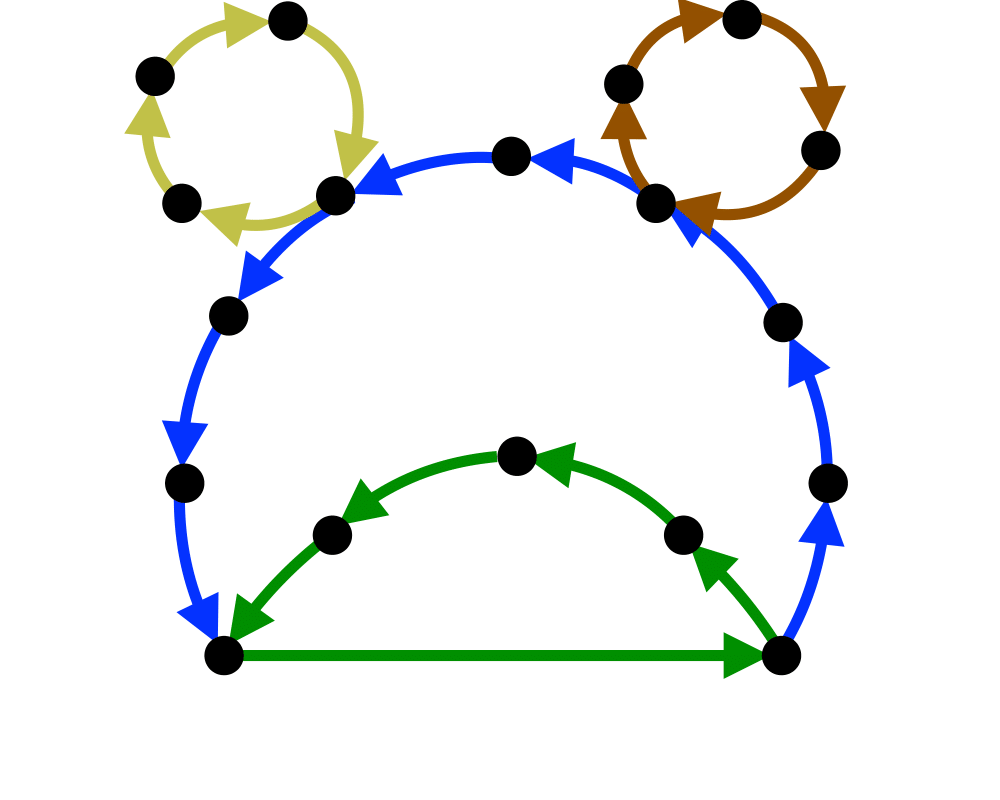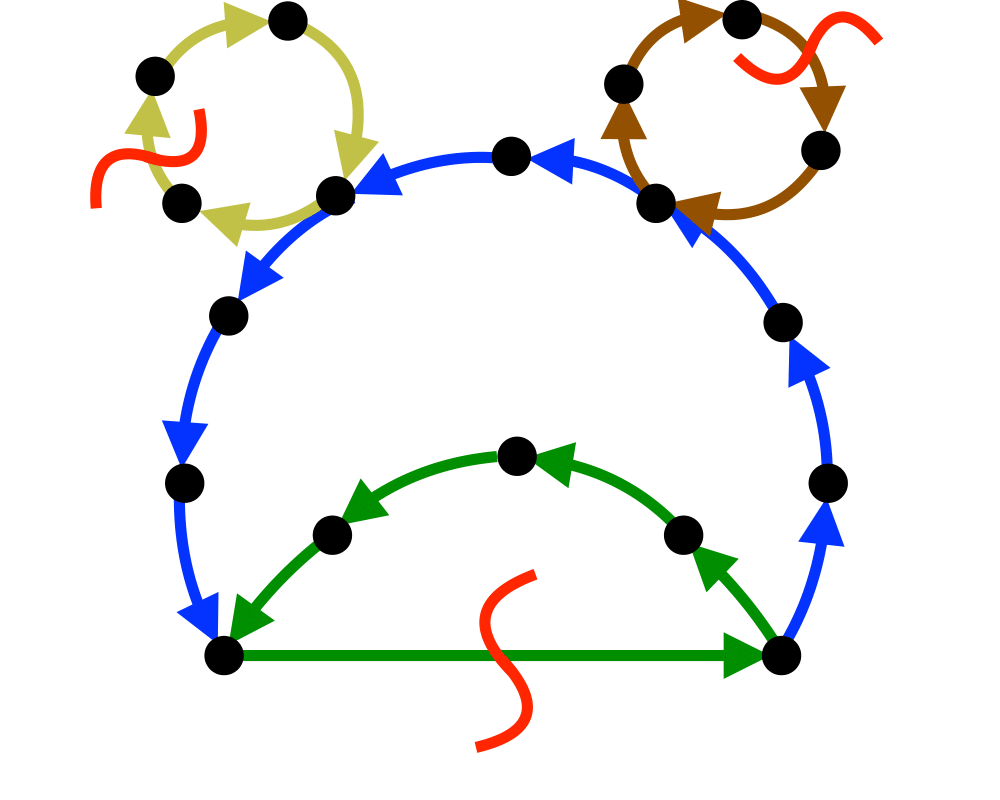# Feedback Edge/Vertex SetInputOutput

Input Description: A (directed) graph $$G=(V,E)$$.
Problem: What is the smallest set of edges $$E'$$ or vertices $$V'$$ whose deletion leaves an acyclic graph?

Excerpt from The Algorithm Design Manual: Feedback set problems arise because many algorithmic problems are much easier or much better defined on directed acyclic graphs than on arbitrary digraphs. Topological sorting can be used to test whether a graph is a DAG, and if so, to order the vertices so as to respect the edges as precedence scheduling constraints. But how can you design a schedule if there are cyclic constraints, such as $$A$$ must be done before $$B$$, which must be done before $$C$$, which must be done before $$A$$?

By identifying a feedback set, we identify the smallest number of constraints that must be dropped so as to permit a valid schedule. In the feedback edge (or arc) set problem, we drop precedence constraints (job $$A$$ must come before job $$B$$). In the feedback vertex set problem, we drop entire jobs and any associated constraints. It is also referred to in the literature as the maximum acyclic subgraph problem.

Go To Main Page